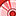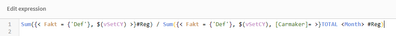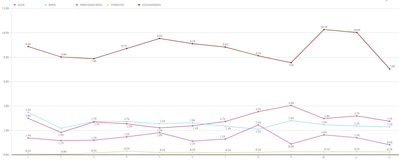# App Development

Announcements
QlikWorld happening right now! You can still join: REGISTER NOW
cancel
Showing results for
Did you mean:Contributor II

## Cumulative Percentage - Line Chart

I have a line chart with monthly values (percentages). I try to make the same chart, but with cumulative values but without success. Could you help me with modification my expression?

Expression and chart below are for monthly values. There are no all carmakers (I have active filter for carmakers because of clear arrangement), but percentage is counting from all.
vSetCY = current year
#Reg = number of sales
Fakt=dimension

Thank you

*let me know, if everything is clear or obscure...Sum({< Fakt = {'Def'}, \$(vSetCY) >}#Reg) / Sum({< Fakt = {'Def'}, \$(vSetCY), [Carmaker]= >}TOTAL <Month> #Reg)I made an attempt to do it, but this is only cumulative without percentage:

=(Aggr(RangeSum(Above(Sum({< Fakt = {'Def'}, \$(vSetCY), [Carmaker]= >}#Reg), 0, RowNo())), [Carmaker], Month))Labels (4)

• ### percentage

1 Solution

Accepted SolutionsContributor II
Author

I get it. It works for me perfectly:

aggr(RangeSum(Above(Sum({< Fakt = {'Def'}, \$(vSetCY) >}#Reg), 0, RowNo())), Fakt, [Carmaker], Month) / aggr(RangeSum(Above(Sum({< Fakt = {'Def'}, \$(vSetCY), [Carmaker] >}Total <Month> #Reg), 0, RowNo())), [Carmaker], Month)